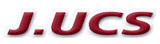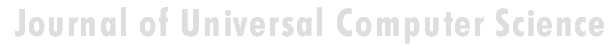Search
 Submission Procedure share: |available in:   PDF (228 kB) PS (183 kB)

get:
 Similar Docs BibTeX Write a comment
get:

DOI:   10.3217/jucs-013-11-1628

### On BCK Algebras - Part I.a: An Attempt to Treat Unitarily the Algebras of Logic. New Algebras

Afrodita Iorgulescu (Academy of Economic Studies, Romania)

Abstract: Since all the algebras connected to logic have, more or less explicitely, an associated order relation, it follows that they have two presentations, dual to each other. We classify these dual presentations in "left" and "right" ones and we consider that, when dealing with several algebras in the same research, it is useful to present them unitarily, either as "left" algebras or as "right" algebras. In some circumstances, this choice is essential, for instance if we want to build the ordinal sum (product) between a BL algebra and an MV algebra. We have chosen the "left" presentation and several algebras of logic have been redefined as particular cases of BCK algebras.

We introduce several new properties of algebras of logic, besides those usually existing in the literature, which generate a more refined classification, depending on the properties satisfied. In this work (Parts I-V) we make an exhaustive study of these algebras - with two bounds and with one bound - and we present classes of finite examples, in bounded case.

In this Part I, divided in two because of its length, after surveying chronologically several algebras related to logic, as residuated lattices, Hilbert algebras, MV algebras, divisible residuated lattices, BCK algebras, Wajsberg algebras, BL algebras, MTL algebras, WNM algebras, IMTL algebras, NM algebras, we propose a methodology in two steps for the simultaneous work with them (the first part of Part I).

We then apply the methodology, redefining those algebras as particular cases of reversed left-BCK algebras. We analyse among others the properties Weak Nilpotent Minimum and Double Negation of a bounded BCK(P) lattice, we introduce new corresponding algebras and we establish hierarchies (the subsequent part of Part I).

Keywords: BCK algebra, BCK(P) lattice, BL algebra, Hertz algebra, Heyting algebra, Hilbert algebra, Hájek(P) algebra, IMTL algebra, MTL algebra, MV algebra, NM algebra, R0 algebra, WNM algebra, Wajsberg algebra, divisible BCK(P) lattice, generalized Wajsberg algebra, generalized-BL algebra, generalized-MV algebra, pocrim, residuated lattice, t-norm, weak-BL algebra

Categories: F.4.1